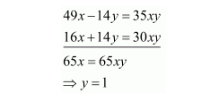# Solve the following systems of equations:

Question:

Solve the following systems of equations:

$\frac{7 x-2 y}{x y}=5$

$\frac{8 x+7 y}{x y}=15$

Solution:

The given equations are:

$\frac{7 x-2 y}{x y}=5$

$7 x-2 y=5 x y$....(i)

$\frac{8 x+7 y}{x y}=15$

$8 x+7 y=15 x y$$\ldots($ ii $)$

Multiply equation $(i)$ by 7 and equation $(i i)$ by 2 , add both equations we getPut the value of $y$ in equation $(i)$, we get

$7 x-2 \times 1=5 x \times 1$

$\Rightarrow 2 x=2$

$\Rightarrow x=1$

Hence the value of $x=1$ and $y=1$